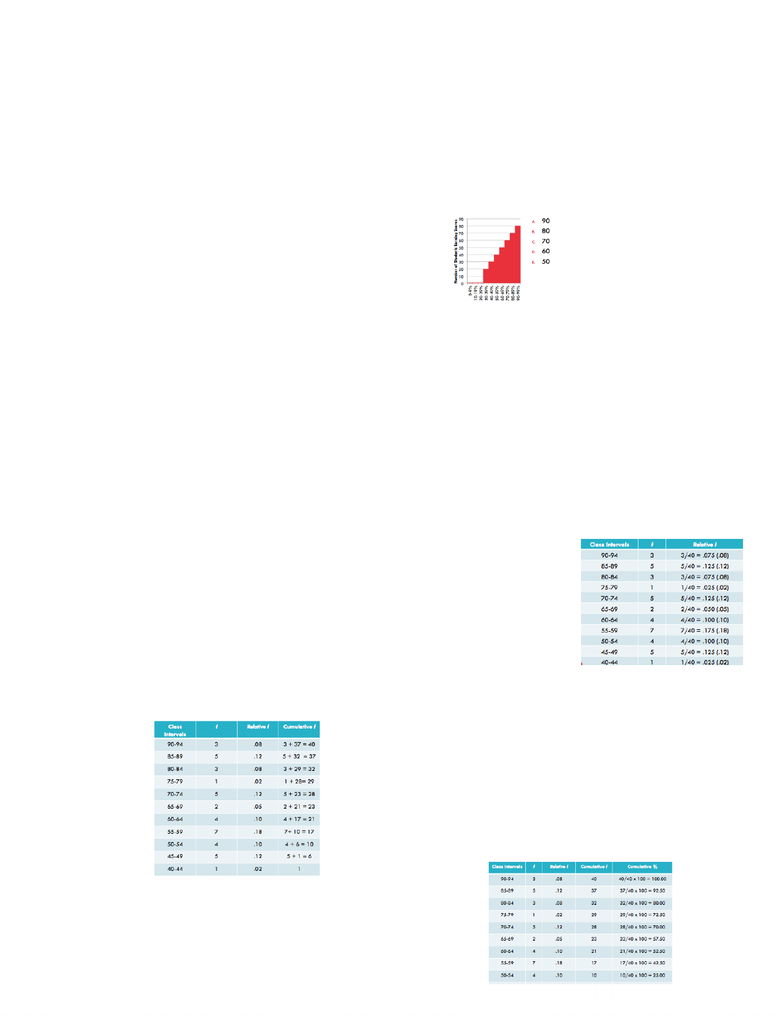# PSY201H1 Lecture Notes - Cumulative Frequency Analysis, Level Of Measurement, Frequency Distribution

30 views4 pages
School
UTSG
Department
Psychology
Course
PSY201H1Sept 20
Learning objectives:
- Use 5 steps to calculate frequency distribution of scores sorted into intervals
- Distinguish among 3 types of frequency distributions (calculation and interpretation)
- Distinguish between 4 types of graphs
- Interpret and calculate percentile score
- Distinguish between percentile and percentile mark
- Interpret and calculate mean, median, and mode
- List and describe all 5 properties that make mean special
- Explain how measures of central tendency change when shape of distribution changes
How many students fit within 60-69% range? E.
A. How do we calculate a frequency distribution using intervals?
(i) Find the range of scores
a. Range = Highest score-Lowest score
b. 93-42 =51
(ii) Determine interval width
a. Assume we want ~10 intervals
b. Determine i (interval width)
c. i = Range/Number of intervals
d. Ex. i=51/10=5.1
e. Round i to same number of decimal places as in raw data
(iii) List the limits of each class interval
a. Begin with lowest interval
i. Lowest interval must contain lowest score
ii. Lower limit of lowest interval must be evenly divisible by i
b. Ex. Lowest score is 42. What is the lowest interval? B
i. A. 38-43
ii. B. 40-44
iii. C. 42-47
iv. E. 42-48
(iv) Tally up the scores in each interval and enter amount in frequency (f) column
B. 3 Kinds of Frequency Distributions
- Relative Frequency Distribution
o Indicates proportion of total number of scores that occur in each interval
o Relative f = f/N
o Ex. If N=40, what is the relative frequency of 85-89 interval?
5/40=.125 (.12)
- Cumulative Frequency Distribution
o Number of scores that fall below the upper real limit of each interval
o Starting on the bottom interval add the frequency of interval to frequencies of all intervals below it
- Cumulative Percentage Distribution
o % of scores that fall below upper real limit of each interval
o cum % = (cum f/n)x100
Unlock document

This preview shows page 1 of the document.
Unlock all 4 pages and 3 million more documents.

## Document Summary

Use 5 steps to calculate frequency distribution of scores sorted into intervals. Distinguish among 3 types of frequency distributions (calculation and interpretation) Explain how measures of central tendency change when shape of distribution changes. List and describe all 5 properties that make mean special. E: how do we calculate a frequency distribution using intervals? (i) (ii) (iii) Find the range of scores: range = highest score-lowest score, 93-42 =51. Determine interval width: assume we want ~10 intervals, determine i (interval width, ex. i=51/10=5. 1, round i to same number of decimal places as in raw data. List the limits of each class interval: begin with lowest interval i = range/number of intervals, lowest interval must contain lowest score, lower limit of lowest interval must be evenly divisible by i, ex. Tally up the scores in each interval and enter amount in frequency (f) column: 3 kinds of frequency distributions.